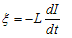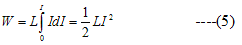# Energy in inductor

## Energy in an inductor

• Changing current in an inductor gives rise to self induced emf which opposes changes in the current flowing through the inductor.
• This self inductance thus plays the role the inertia and it is electromagnetic analogue of mass in mechanics.
• So a certain amount of work is required to be done against this self induced emf for establishing the current in the circuit.
• In order to do so, the source supplying current in a circuit must maintain Potential difference between its terminals which is done by supplying energy to the inductor.
• Power supplied to the inductor is given by relation
P=ξI                      ---(4)
whereL is Self inductance and
dI/dt is rate of change of current I in the circuit.
• Energy dW supplied in time dt would be
dW=Pdt
=LI(dI/dt) dt
=LIdI
and total energy supplied while current I increases from o to a final value I is• Once the current reaches its final value and becomes steady ,the power input becomes zero.
• The energy so far supplied to the inductor is stored in it as a form of potential energy as long as current is maintained.
• When current in circuit becomes zero, the energy is returned to the circuit which supplies it.